Thursday, March 6, 2014

Exceptions to Continuity 3

This is the final part of a three-part post. For the first, see here.

In the previous post, it was stated that every function has an Fσ set of discontinuities. We cannot prove this fact, since the proof is beyond the scope of this blog, but we can demonstrate its converse, which is also true, namely that for any Fσ set S, there exists a function f: RR (a real-valued function defined on all reals) with a set of discontinuities S.

Expand S into its subsets in the following way: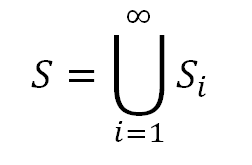where each Si is closed (this is just the definition of an Fσ set, i.e. a countable union of closed sets). We wish to define this set as an Fσ set in a slightly different way as a countable union of closed sets. We define a new series of sets Tj, where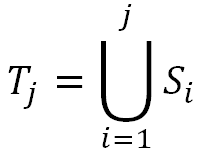In other words, T1 = S1, T2 = S1S2, and in general the Tj is the union of the first j sets of the collection of S-sets, {Si|iZ+}. Note that each Tj is a closed set, being a union of a finite number of closed sets (since j is finite), and that the union of all of the Tj is the same as the union of all of the Si, since each Tj is simply formed from a union of the Si. However, this new collection of sets possesses a useful feature in constructing our desired function f: each Tj is contained within the next member of the sequence Tj + 1.

Before defining the function itself, we need one more collection of sets based off of the Tj. These sets, for each j, essentially carry information about the differences between consecutive sets among the Tj. Symbolically, they are defined in the following way:What this means is that Uj contains those real numbers which are contained in the set difference of Tj and Tj - 1, or the set of numbers in Tj but not Tj - 1 (note in fact, that this set difference might be empty; we will return to this later). Note also that for j = 1, the definition of U1 makes use of a set "T0". We define this to be the empty set. Finally, among the real numbers contained in the set difference, only those which are on the boundary of the set (the condition preceding the "or" symbol, ∨) or are rational (the second condition, which states that such numbers are contained within the intersection of the set difference and the rational numbers, Q) are in Uj. The importance of these conditions will be made clear, but first we must finally define our long-sought function f: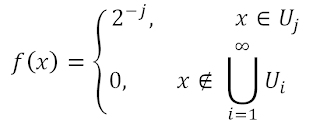Thus f takes a different constant value on each of the Uj, namely 2-j. This definition is well-defined because no two Uj intersect. If some member x of Um were a member of Un for n > m, then x would have to be contained in the set difference Tn - Tn - 1. However, since mn - 1, x must also be within Tn - 1, since the T's are nested. This is a contradiction, so the Uj are disjoint. Finally, the function f is defined as 0 outside of all of the Uj. Next, to illustrate how this definition works and is applied to sets, we consider a few examples.

Let S be the set {1/5,2,π}. To illustrate the concepts above, we initially assign S1 = {1/5}, S2 = {2}, S3 = {π}, and Sn = Ø for n ≥ 4 (note that this is not the only way to divide S into sets Si and that different divisions may yield different functions with the same discontinities). Clearly the union of the Si is indeed S. Next, T1 = S1 = {1/5}, T2 = S1S2 = {1/5,2}, T3 = S1S2S3 = {1/5,2,π}, and Tn = {1/5,2,π} for n ≥ 4, since the Si are empty above i = 3 and empty sets do not contribute to a union. Also, by definition, T0 is the empty set. Now we shall determine what form the Uj take. U1 takes elements from the set difference T1 - T0 = {1/5} - Ø = {1/5}. Since 1/5 is rational, it satisfies at least one of the additional conditions above and is indeed in U1. There are no other elements in T1 - T0, so U0 = {1/5}. Similarly, T2 - T1 contains one member, 2, and since it is rational, it is also the sole member of U2. Finally, T3 - T2 = {π}. Though π is not rational and so is not a member of (T3 - T2)∩Q, it still is contained within U3 because it is on the boundary of the set difference (the boundary of a set containing a single point is merely the same set). Thus U3 = {π}.

Using these facts, our previous definitions yield the following formula for f:This formula yields the graph below: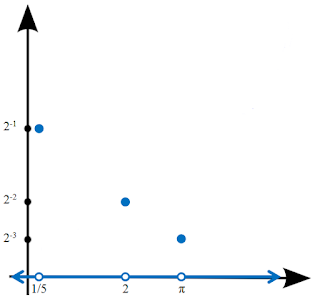Clearly this function has exactly our desired set S = {1/5,2,π} as its set of discontinuities. Note also the importance of the condition that those elements on the boundary of the set difference Ti - Ti - 1 are in Ui, as this was crucial in having π as a discontinuity, since it is irrational.

For a more intricate example, we now allow the set S to be the half-open interval (0,1]. S is Fσ because it is the union of the sets [1/2,1], [1/3,1/2], [1/4,1/3],..., each of which is closed. We therefore let Si = [1/(i + 1), 1/i] for every positive integer i. Clearly each number between 0 and 1 is contained in one of these sets, and 1 is contained in S1. However, the union of the infinite collection of sets is (0,1] because it does not include 0; if 0 were a member of the union, it would have to be a member of some Si. However, 0 is strictly less than 1/i for any positive integer i, and therefore cannot be in the union of the Si.

From these definitions for the Si, we see that T1 = S1 = [1/2,1], T2 = S1S2 = [1/3,1], and in general, Tj = [1/(j + 1),1]. Each set difference Tj - Tj - 1 is of the form [1/(j + 1),1/j), except for T1 - T0, which equals [1/2,1] and includes its right endpoint, since T0 is the empty set. As before, we select certain elements from each set difference to form the Uj, namely those which are rational (the boundary condition offers nothing new, since ∂(Tj - Tj - 1) = ∂[1/(j + 1),1/j) = {1/(j + 1)}, and 1/(j + 1) is rational). Thus each Uj contains exactly the the rationals between 1/(j + 1) and 1/j, including 1/(j + 1) but not including 1/j. Restricting our attention briefly to just one of these intervals, we see, for example in the vicinity of 2/3, which is in U1, that our function f will assume positive values at rationals and a value of zero at irrationals, since no irrational is a member of any Uj for this function. Our entire function will then consist of several smaller "copies" of the nowhere continuous Dirichlet function, which is 1 at rationals and 0 at irrationals. Before presenting a visualization of this function, we give its definition:The image below "graphs" this function. Due to the Dirichlet construction, the function jumps infinitely many times within every interval between 0 and 1. Therefore, when the function is split among the rationals and irrationals, the function's value at the rationals is given as a short-dashed line, and the function's value at the irrationals a long-dashed line. Note that at the value 2/3 below, the long-dashed line is at 0 and the short-dashed line at 2-1. Additionally, the segments on which the rationals are situated continued to shrink and move towards 0 as one approaches the origin, as indicated by the ellipsis.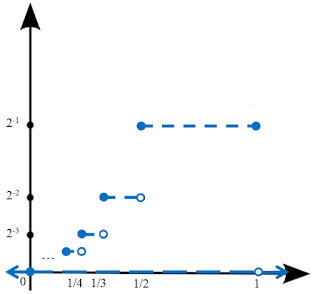It is easy to see that the function f is discontinuous on the entire interior of the unit interval, (0,1), because member of this set is surrounded arbitrarily closely by rational numbers, for which f is nonzero, and irrational numbers, for which f is zero. Additionally, since f(1) = 2-1, and f(x) = 0 for x > 1, f is additionally discontinuous at 1. However, to see that f is indeed discontinuous at exactly the set S, we must show the the function is not discontinuous, that is, continuous, at x = 0.

Consider the behavior of f(x) as x approaches 0. Given an arbitrarily small positive number ε, we can find a positive integer n such that 2-n < ε. As we know, on the interval [0,1/n), the largest value f can take is 2-n. Thus we have that for |x| < 1/n = δ, f(x) will be less than ε. Thus f is continuous at 0, and f has all the desired properties.

We have seen, for two examples, that the process above does yield a function f with discontinuities on exactly the members of a given Fσ set. After the above motivational examples, we finish the post by proving that this fact holds in the general case.

Let S be an Fσ set, f its corresponding function by the above scheme, and let the {Tj} be defined as above. We consider first the case of a point belonging to one of the set differences Tj - Tj - 1. If a point x is in the interior of this set difference f is discontinuous at x for the same reasons that the Dirichlet function is, since f behaves in the same manner as the Dirichlet function on the interiors of the set differences. If x is on the boundary of a set difference, f(x) = 2-j. However, neighboring points outside of the set difference must differ from this value by at least 2-(j + 1), since the closest possible value to 2-j that the function can take outside of Tj - Tj - 1 is 2-(j + 1), namely on the set difference Tj + 1 - Tj. Thus f is discontinuous at x. We have proven that f is discontinuous at every point of every set difference of the Tj. Since the union of the {Tj} is S, we see that S is exactly the union of all the set differences of the {Tj}. Thus f is discontinuous on S.

To finish the proof, we must show that f always continuous outside S. Let x now be any point outside of S, and therefore outside of every set difference. Since each Tj is closed, it contains its boundary, so x cannot be on the boundary of any Tj. Thus there is some neighborhood surrounding the point x that does not contain any member of Tj for some fixed value of j. For example, in the second example above, any set containing 0 in its interior contains part of a set Tj for some j, since the left endpoints of the {Tj} in that example, 1/(j + 1), become arbitrarily small. However, for any fixed Tj, say T3 = [1/4,1], we can choose a neighborhood of 0, for example (-1/5,1/5) which excludes all the {Tj} up to T3. This is the most difficult subtlety of the proof. Having found a neighborhood U of x (in the example, 0) that excludes the first n of the {Tj}, we note that the function must take values less than 2-n on U. Since this can be done for any finite n, we see that the function approaches 0 as one approaches x and that f is continuous at x. Therefore f is discontinuous on exactly the set S.

The above series describes a complete classification of discontinuities for real-valued functions. Also, this and related results give additional insight into the structure of real numbers, for example the distinction between rational numbers, on exactly which set a function can be discontinuous, and the irrational numbers, which are not Fσ. Such a classification demonstrates the power of mathematical techniques, as it provides simple conditions on all of the strange functions and sets which can exist on the real numbers.

Sources: Sources: Counterexamples in Analysis by Bernard R. Gelbaum and John M. H. Olmsted, Froda's Theorem on Wikipedia, http://holdenlee.wordpress.com/2010/04/26/can-a-function-be-continuous-only-on-rationals/, http://samjshah.com/2009/10/03/sin1x/,